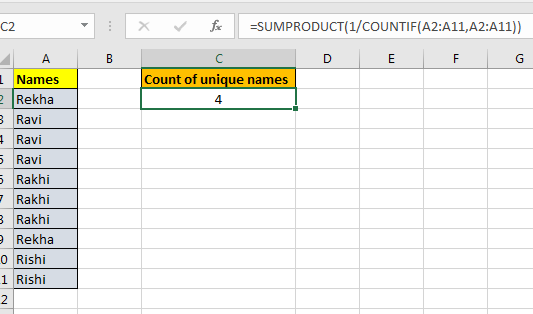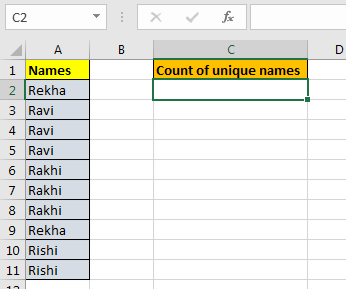# How to Count Unique Values In Excel

We will use SUMPRODUCT and COUNTIF function to count unique values in a range.Generic Formula to Count Unique Values:

=SUMPRODUCT(1/COUNTIF(range,range))

#### Excel Unique Count Example

Lets say we have name list. Now this list may contain duplicate values. How can we count unique names only. See below table.Write this Unique Count formula anywhere on sheet. And you will have unique count of items in given range.

=SUMPRODUCT(1/COUNTIF(A2:A11,A2:A11))#### Explanation:

• COUNTIF(A2:A11,A2:A11) : This part will return an array of the count of each element in the cell. If you select this part of formula and press F9, you will see the array. If “Radha” occurs two times and “Reena” 3 then the array will be. {2,2,3,3,3}.In this example it is {2;3;3;3;3;3;3;2;2;2}.
• 1/COUNTIF(A2:A11,A2:A11) : Here we are dividing 1 by each element of array returned by COUNTIF(A2:A11,A2:A11). It will again return an array of fractions. If array returned by countif function is {2,2,3,3,3} then the fraction array will be {0.5,0.5,0.333,0.333,0.333}. If we some them then we will get {1,1}. 1 for each element in the array. For our example, it is {0.5;0.333;0.333;0.333;0.333;0.333;0.333;0.5;0.5;0.5}.

Still with me? OK, we are almost there.

• SUMPRODUCT(1/COUNTIF(A2:A11,A2:A11)) : It will sum the array provided by 1/COUNTIF(A2:A11,A2:A11), which will be the count of unique values in range.

I don’t know if I was clear enough to make you understand the functionality of this unique count formula but one thing I am sure of that this works fantastically. Let me know in the comments if it was helpful or not.

Related Articles:

Excel Formula to Extract Unique Values From a List

How to Get All Matches in Different Columns

How To Count Unique Values in Excel With Criteria?

How To Count Unique Text in Excel

Popular Articles:

50 Excel Shortcuts to Increase Your Productivity

How to use the VLOOKUP Function in Excel

How to use the COUNTIF function in Excel

How to use the SUMIF Function in Excel

Terms and Conditions of use

The applications/code on this site are distributed as is and without warranties or liability. In no event shall the owner of the copyrights, or the authors of the applications/code be liable for any loss of profit, any problems or any damage resulting from the use or evaluation of the applications/code.